# How to Calculate and Solve for Shielding Factor | Polymer & Textile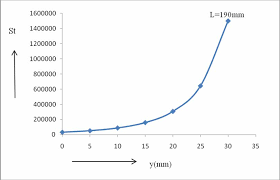The image above represents shielding factor.

To compute for shielding factor, two essential parameter are needed and these parameters are coil radius (Rs) and shielding depth (L).

The formula for calculating the shielding factor:

ε = Rs / L

Where:

ε = Shielding Factor
L = Shielding Depth

Let’s solve an example;
Find the shielding factor when the coil radius is 11 and the shielding depth is 21.

This implies that;

Rs = Coil Radius = 11
L = Shielding Depth = 21

ε = Rs / L
ε = 11 / 21
ε = 0.52

Therefore, the shielding factor is 0.52.

Calculating for the Coil Radius when the Shielding Factor and the Shielding Depth is Given.

Rs = ε x L

Where;

ε = Shielding Factor
L = Shielding Depth

Let’s solve an example;
Given that the shielding factor is 30 and the shielding depth is 5. Find the coil radius?

This implies that;

ε = Shielding Factor = 30
L = Shielding Depth = 5

Rs = ε x L
Rs = 30 x 5
Rs = 150

Therefore, the coil radius is 150.

Calculating for the Shielding Depth when the Shielding Factor and the Coil Radius is Given.

L = Rs / ε

Where;

L = Shielding Depth
ε = Shielding Factor

Let’s solve an example;
With a shielding factor of 24 and a coil radius of 14. Find the value of the shielding depth?

This implies that;

ε = Shielding Factor = 24
Rs = Coil Radius = 14

L = Rs / ε
L = 14 / 24
L = 0.58

Therefore, the shielding depth is 0.58.

Nickzom Calculator – The Calculator Encyclopedia is capable of calculating the shielding factor.

To get the answer and workings of the shielding factor using the Nickzom Calculator – The Calculator Encyclopedia. First, you need to obtain the app.

You can get this app via any of these means:

To get access to the professional version via web, you need to register and subscribe for NGN 1,500 per annum to have utter access to all functionalities.
You can also try the demo version via https://www.nickzom.org/calculator

Apple (Paid) – https://itunes.apple.com/us/app/nickzom-calculator/id1331162702?mt=8
Once, you have obtained the calculator encyclopedia app, proceed to the Calculator Map, then click on Polymer & Textile under Engineering.Now, Click on Shielding Factor under Polymer & Textile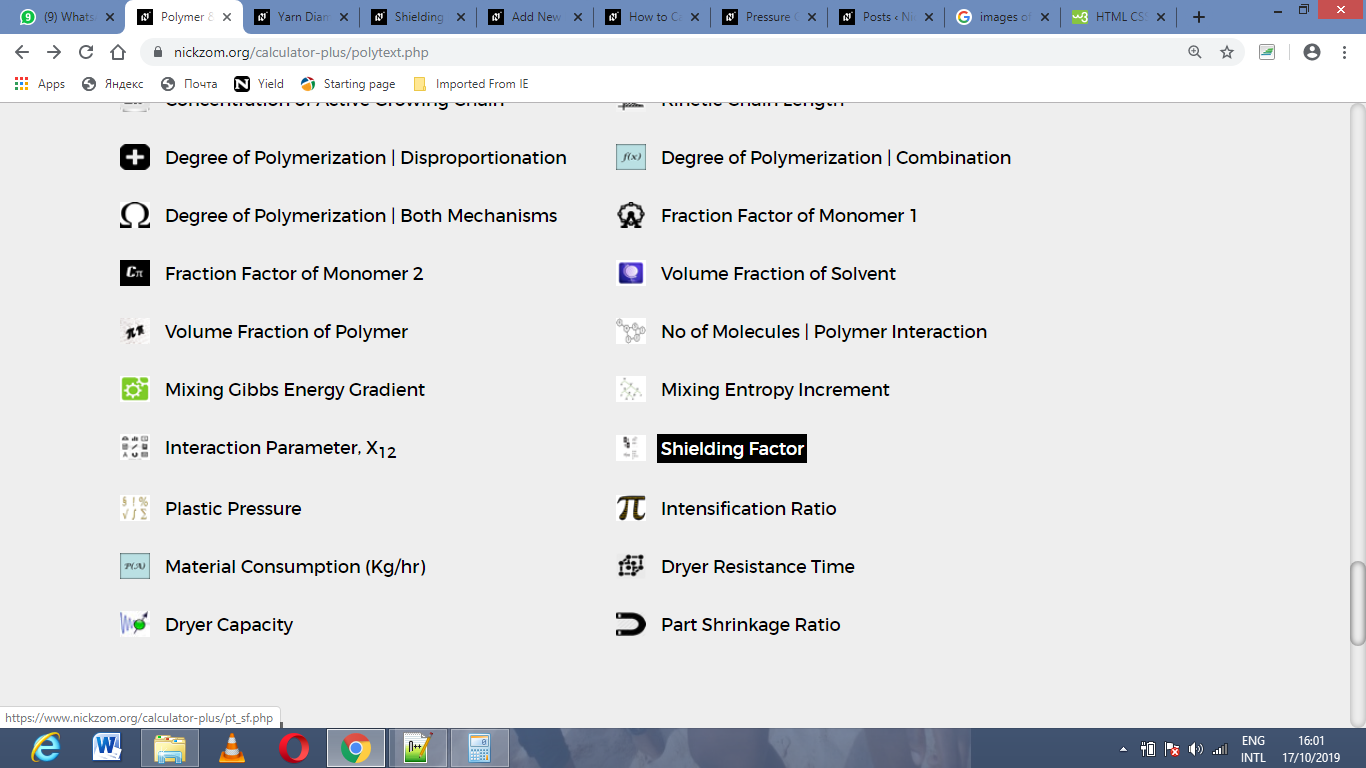The screenshot below displays the page or activity to enter your values, to get the answer for the shielding factor according to the respective parameters which is the coil radius (Rs) and shielding depth (L).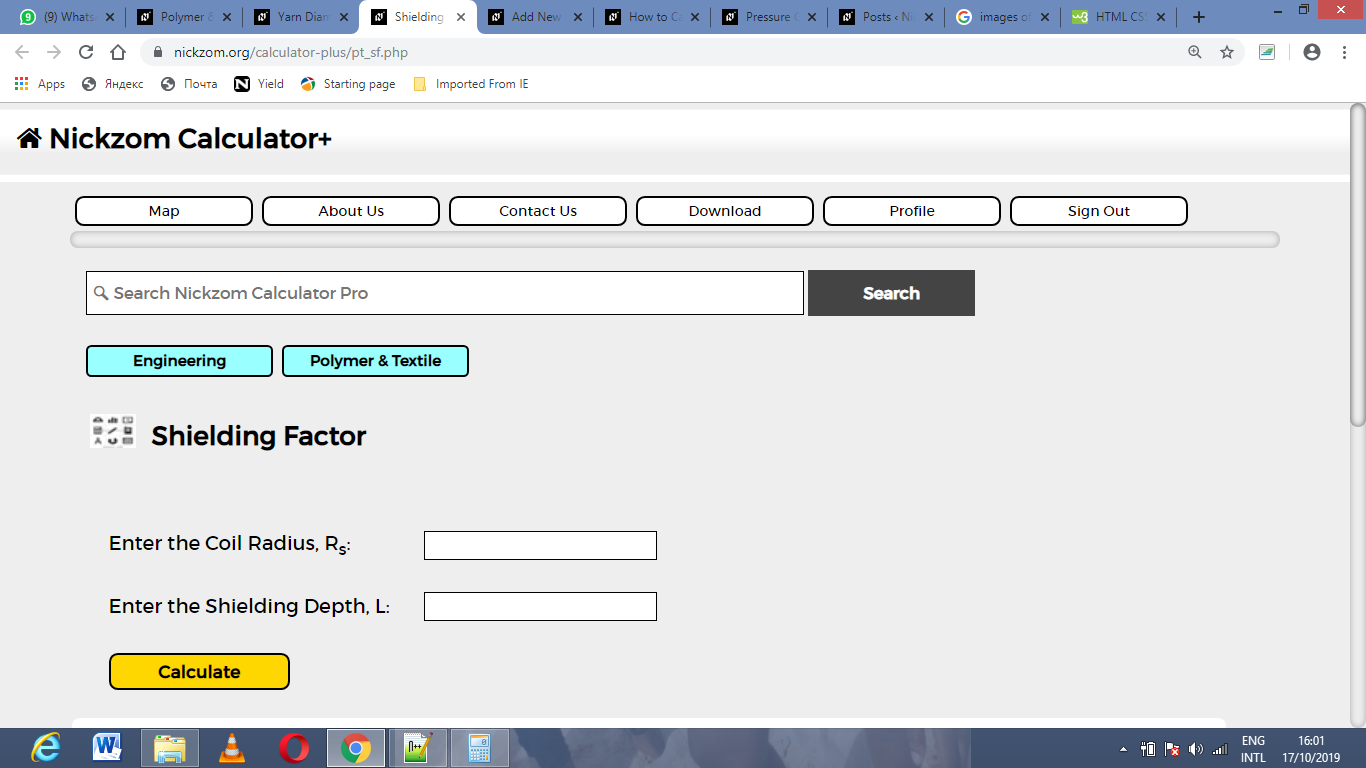Now, enter the values appropriately and accordingly for the parameters as required by the coil radius (Rs) is 11 and shielding depth (L) is 21.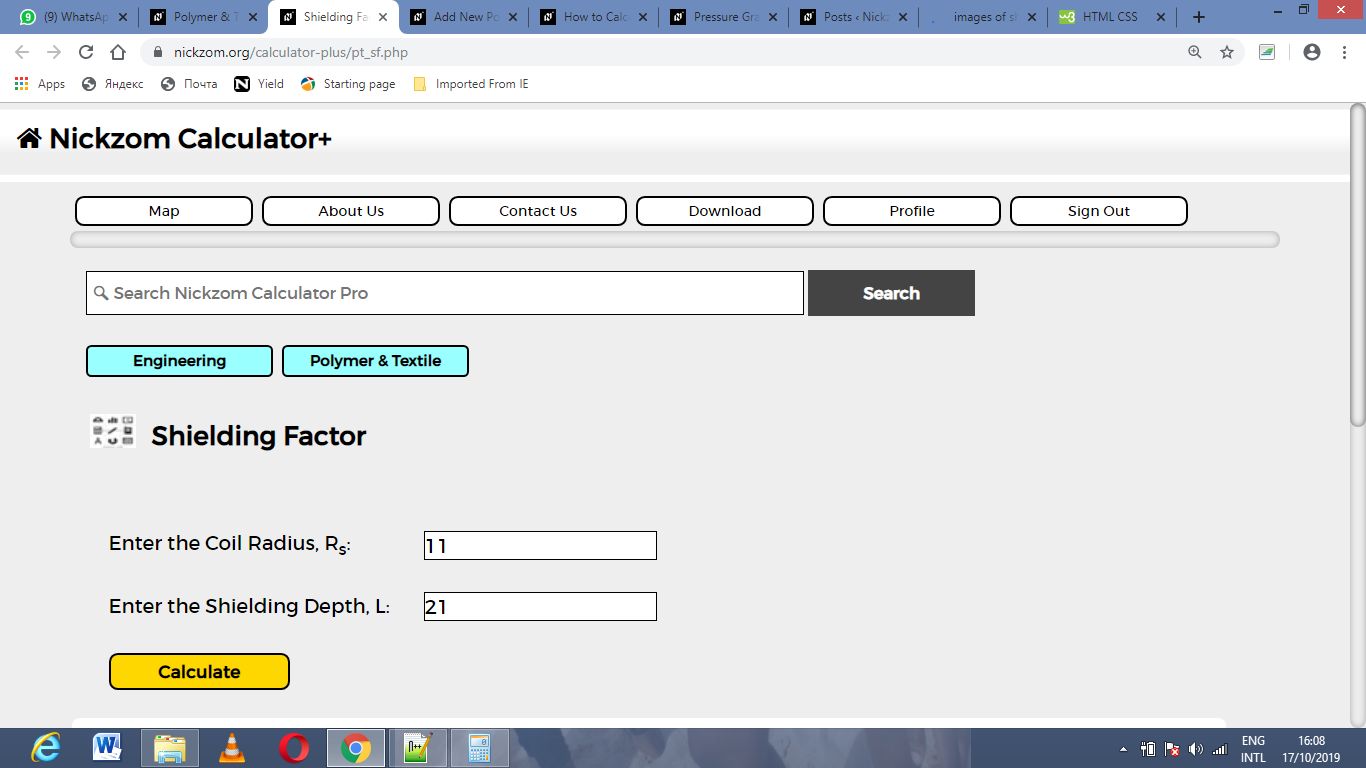Finally, Click on Calculate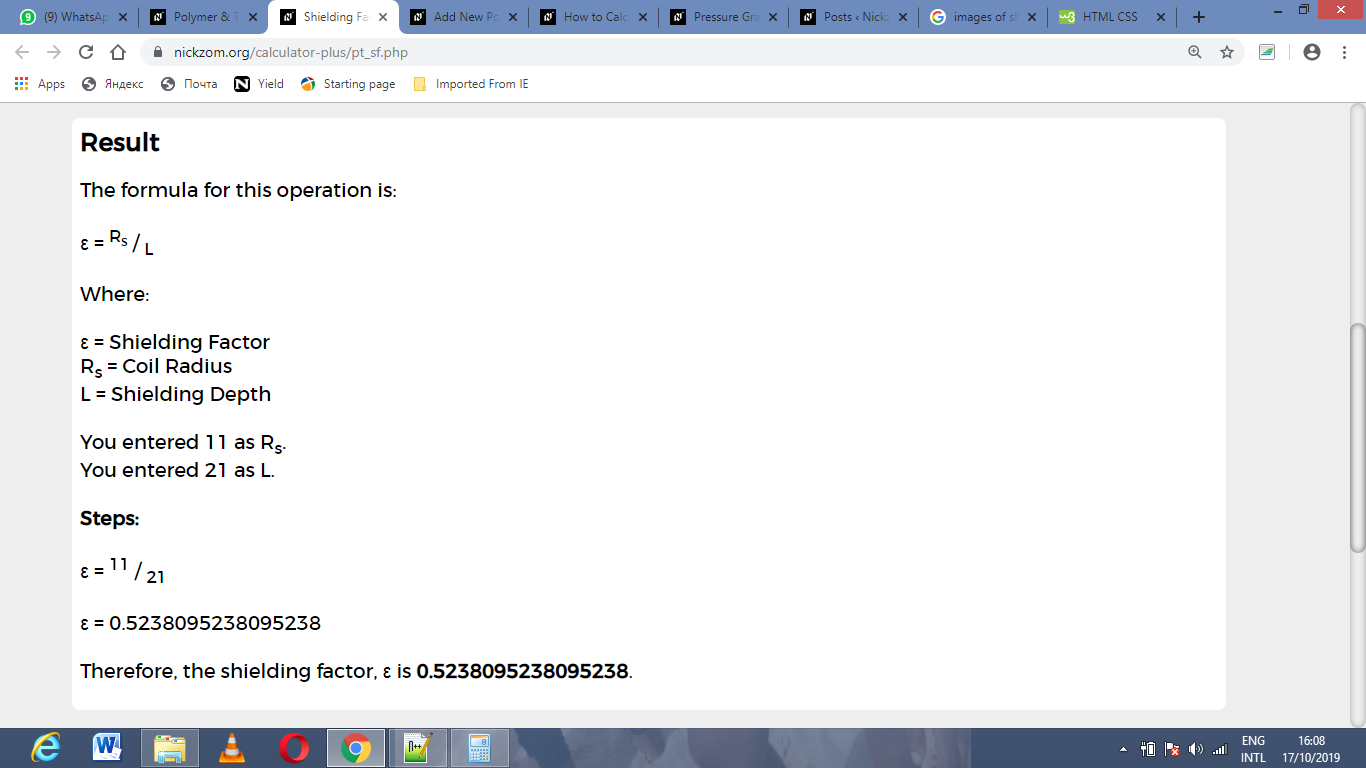As you can see from the screenshot above, Nickzom Calculator– The Calculator Encyclopedia solves for the shielding factor and presents the formula, workings and steps too.